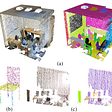# Description

An ML model that classifies yoga pose into 4 most famous asanas namely downward dog, plank pose, tree pose, goddess pose, and warrior-2 pose using Mediapipe Blazepose for feature extraction.

# DataSet

Dataset is a combined Dataset of :

# Rescaling images

Images are first resized to reduce computation.

`DESIRED_HEIGHT = 480DESIRED_WIDTH = 480def resize_2D_array(overall_images):    new_arr_for_outerdirectory = []    for i in range(len(overall_images)):      col = dict[i]      new_array_for_subdirectory = []      for j in range(len(overall_images[i])):        img = overall_images[i][j]        h, w = img.shape[:2]        if h < w:          img = cv2.resize(img, (DESIRED_WIDTH, math.floor(h/(w/DESIRED_WIDTH))))          print(img.shape)                else:          img = cv2.resize(img, (math.floor(w/(h/DESIRED_HEIGHT)), DESIRED_HEIGHT))          print(img.shape)           #appending the image to a new array        new_array_for_subdirectory.append(img)      new_arr_for_outerdirectory.append(new_array_for_subdirectory)    return new_arr_for_outerdirectory`

Gamma correction is a non-linear adjustment to individual pixel values. In image normalization, linear operations are carried out on individual pixels, gamma correction carries out a non-linear operation on the source image pixels, and can cause saturation of the image to bealtered.

`#for brightness improvementdef gammaCorrection(src, gamma):    invGamma = 1 / gamma     table = [((i / 255) ** invGamma) * 255 for i in range(256)]    table = np.array(table, np.uint8)     return cv2.LUT(src, table)def isbright(image, dim=10):    # Resize image to 10x10    image = cv2.resize(image, (dim, dim))    # Convert color space to LAB format and extract L channel    L, A, B = cv2.split(cv2.cvtColor(image, cv2.COLOR_BGR2LAB))    # Normalize L channel by dividing all pixel values with maximum pixel value    L = L/np.max(L)    # Return True if mean is greater than thresh else False    return np.mean(L)def changeBrightness(image):  if (isbright(image) < 0.5):    gammaImg = gammaCorrection(image, 1)  elif (isbright(image) > 0.85):    gammaImg = gammaCorrection(image, 0.75)  else:    gammaImg = image  return gammaImgdef improve_brightness(overall_images):    new_arr = []    for i in range(len(overall_images)):      col = dict[i]      new_array_1 = []      for j in range(len(overall_images[i])):        img = changeBrightness(overall_images[i][j])        #appending the image to a new array        new_array_1.append(img)      new_arr.append(new_array_1)    return new_arr`

• Adjusts image contrast by its histogram.
• To enhance contrast, spreads out intensity range of image.
• This allows the image’s areas with lower contrast to gain a higher contrast.
`# import the neccessasry libraryfrom skimage.exposure import is_low_contrastdef histogram_equalization(img_in):# segregate color streams    b,g,r = cv2.split(img_in)    h_b, bin_b = np.histogram(b.flatten(), 256, [0, 256])    h_g, bin_g = np.histogram(g.flatten(), 256, [0, 256])    h_r, bin_r = np.histogram(r.flatten(), 256, [0, 256])# calculate cdf        cdf_b = np.cumsum(h_b)      cdf_g = np.cumsum(h_g)    cdf_r = np.cumsum(h_r)    # mask all pixels with value=0 and replace it with mean of the pixel values     cdf_m_b = np.ma.masked_equal(cdf_b,0)    cdf_m_b = (cdf_m_b - cdf_m_b.min())*255/(cdf_m_b.max()-cdf_m_b.min())    cdf_final_b = np.ma.filled(cdf_m_b,0).astype('uint8')      cdf_m_g = np.ma.masked_equal(cdf_g,0)    cdf_m_g = (cdf_m_g - cdf_m_g.min())*255/(cdf_m_g.max()-cdf_m_g.min())    cdf_final_g = np.ma.filled(cdf_m_g,0).astype('uint8')    cdf_m_r = np.ma.masked_equal(cdf_r,0)    cdf_m_r = (cdf_m_r - cdf_m_r.min())*255/(cdf_m_r.max()-cdf_m_r.min())    cdf_final_r = np.ma.filled(cdf_m_r,0).astype('uint8')    # merge the images in the three channels    img_b = cdf_final_b[b]    img_g = cdf_final_g[g]    img_r = cdf_final_r[r]      img_out = cv2.merge((img_b, img_g, img_r))# validation    equ_b = cv2.equalizeHist(b)    equ_g = cv2.equalizeHist(g)    equ_r = cv2.equalizeHist(r)    equ = cv2.merge((equ_b, equ_g, equ_r))      return img_out    def improve_contrast(overall_images):    new_arr = []    for i in range(len(overall_images)):      col = dict[i]      new_array_1 = []      for j in range(len(overall_images[i])):                img = overall_images[i][j]        if(is_low_contrast(img, fraction_threshold=0.05, lower_percentile=1, upper_percentile=99, method='linear')):          img = histogram_equalization(img)        #appending the image to a new array        new_array_1.append(img)      new_arr.append(new_array_1)    return new_arrnewarray = improve_contrast(newarray)`

# Sharpening Images

• Edge detector used to compute the second derivatives of an image.
• This determines if a change in adjacent pixel values is from an edge or continuous progression. Laplacian filter kernels usually contain negative values in a cross pattern, centered within the array. The corners are either zero or positive values. The center value can be either negative or positive.
`def sharpenimage(image):  laplacian_var = cv2.Laplacian(image, cv2.CV_64F).var()  if laplacian_var < 100:    kernel = np.array([[0, -1, 0],                      [-1, 5,-1],                      [0, -1, 0]])    sharpened_img = cv2.filter2D(src=image, ddepth=-1, kernel=kernel)  else:    sharpened_img = image  return sharpened_imgdef improve_sharpening(overall_images):    new_arr = []    for i in range(len(overall_images)):      col = dict[i]      new_array_1 = []      for j in range(len(overall_images[i])):        img = sharpenimage(overall_images[i][j])        #appending the image to a new array        new_array_1.append(img)      new_arr.append(new_array_1)    return new_arr`

# Body Segmentation

• Media Pipe Segmentation function is used to blur the background of the image
• The mask has the same width and height as the input image, and contains values in [0.0, 1.0] where 1.0 and 0.0 indicate “human” and “background” pixel respectively.

# Pose Landmarks

• Media pipe blaze pose is used to extract 3D coordinates of 33 joints from the image
• x and y: Landmark coordinates normalized to [0.0, 1.0] by the image width and height respectively.
• z: Represents the landmark depth with the depth at the midpoint of hips being the origin, and the smaller the value the closer the landmark is to the camera.

# Angle Computation

Key angles at ( knee , elbow , shoulder , ankle ) are calculated from the points extracted and labelled with respective Asana name Angle at a joint is given by:

angle = degrees(atan2(y3 — y2, x3 — x2) — math.atan2(y1 — y2, x1 — x2))

`def calculateAngle(landmark1, landmark2, landmark3):     x1, y1, _ = landmark1    x2, y2, _ = landmark2    x3, y3, _ = landmark3     angle = math.degrees(math.atan2(y3 - y2, x3 - x2) - math.atan2(y1 - y2, x1 - x2))        # Check if the angle is less than zero.     if angle< 0:         # Add 360 to the found angle.        angle += 360        return angle`

# ML model results:

Train and test machine learning algorithms (Random Forest, SVC, Decision Tree, KNN, Adaboost, RFC) using the dataframe (csv) generated to find which model best fits.

--

--

--

Love podcasts or audiobooks? Learn on the go with our new app.

## Machine Learning — How it works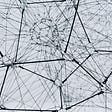## How did I start learning ML? — in Nepal## Kaggle Titanic Challenge: Create New Features Using Extracted Data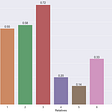## Fruit and Vegetable Detection and Feature Extraction using Instance Segmentation-Part 2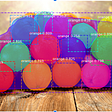## Logistic Regression and Machine Learning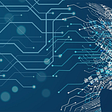## Supervised Learning for Dummies!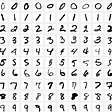## Do You Want To Know How Perceptron Algorithm works Internally## Part 3: Model Testing — Image Classification with YonoHub & Tensorflow V2.0 Series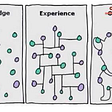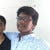## How to enable TensorFlow to use GPU drivers on Windows## How to analyze tweets?## The many uses of a Vector Database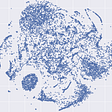## SGPN paper explained